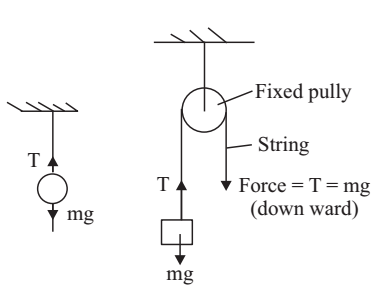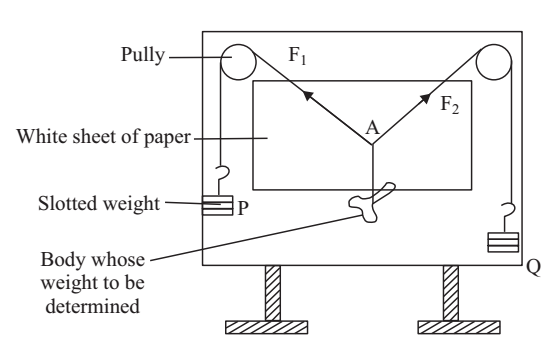# Law of Parallelogram of Vectors Experiment

Experiment: To find the weight of a given body using law of parallelogram of vectors.

According to Newton’s Third Law of motion, tension in a string supporting a body is equal to the weight of the body.The weight due to a body of mass m = mg

Therefore, tension in the string T = mg

A fixed pulley only changes the direction of force and not its value.

Forces are vectors and they cannot be added arithmetically. Resultant force is a single force that produces the same effect as a combination of two or more forces. A body is said to be in equilibrium if the resultant force on it is zero.

Law of parallelogram of vectors: If two vectors acting simultaneously on a particle be represented in magnitude and direction by the two adjacent sides of a parallelogram drawn from a point, then their resultant is completely represented in magnitude and direction by the diagonal of that parallelogram drawn from that point.

F1 and F2 are two forces acting simultaneously on point object at A at an angle θ. They are represented in magnitude and direction by sides AB and AD of the parallelogram ABCD. The diagonal AC will represent R the resultant force.Material Required

Parallelogram law of forces apparatus (Gravesand’s apparatus), plumb line, slotted weights, thin strong thread, white drawing, paper sheet, drawing pins, mirror strip, pencil, set square/ protractor, a body whose weight is to be determined

### How To Perform Experiment

1. Set up the Gravesand’s apparatus with its-board vertical arid stable on a rigid base. Check this with the help of a plumb line.2. Oil the axle of pulley so as to make them move freely.

3. Fix the white drawing sheet on the board with the help of pins.

4. Cut a 1m long thread. Tie the hooks of the slotted weights at its ends.

5. Pass the thread over the two pulleys. The hangers must hang freely and they should not touch the board or pulley or ground.

6. Cut 50 cm long thread. Tie the body whose weight is to be determined at one end of the string.

7. Knot the other end to the centre of 1m thread at A.

8. Adjust the three weights such that the junction A stays in equilibrium slightly below the middle of the paper. The three forces are:

F1 due to slotted weights P.

F2 due to slotted weights Q.

R due to the weight of the body.

F1 = P (slotted weight + Weight of hanger)

F2 = Q (slotted weight + weight of hanger)

Perhaps with a given set of weights P and Q and body of unknown weight you find that central junction A can stay anywhere within a circle. Try to locate the centre of this area and bring the junction A there.

9. To mark the direction of the forces, place the plane mirror strip lengthwise under each thread in turn. Mark two points one on either ends of mirror strip by placing your eye in such a position that the image of the thread in strip is covered by the thread itself. The points should be marked only weights are at rest.

10. Note the value of the weights P and Q. Do not forget to add the weight of the hanger along with each. Find weight of hanger by spring balance.

11. Remove the sheet of paper. Join the marked points to show the direction of forces.

12. Choose a suitable scale to indicate the forces, so as to get a large parallelogram.

From A mark off B such that AB = Q/n and D such that AD = P/n to represent forces due to n the weights and hanger. Here, n grown weight is represented by 1 cm.

The number n should be so chosen that the lengths AB and AD are accommodated in the drawing sheets.

13. Repeat the experiment twice again by changing weights in the hangers. Find the average value of the unknown weight.